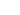# OR Function

This article explains how to use the OR function

# Sample Usage

``OR(QA > 50, QB > 50)``
``OR(QA = 1, QB <> 1, QC > 2)``

# Syntax

``OR(logical_expression1, [logical_expression2, ...])``
• `logical_expression1` - An expression or reference to a cell containing an expression that represents some logical value, i.e. TRUE or FALSE, or an expression that can be coerced to a logical value.

• `logical_expression2, ... -` [ OPTIONAL ] - Additional expressions or references to cells containing expressions representing some logical values, i.e. `TRUE` or `FALSE`, or expressions that can be coerced to logical values.

• The number 0 is logically false; all other numbers (including negative numbers) are logically true.